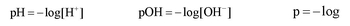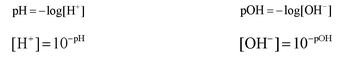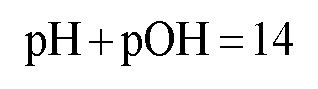Start typing, then use the up and down aroows to select an option from the list.

# GOB Chemistry

Learn the toughest concepts covered in your GOB - General, Organic, and Biological Chemistry class with step-by-step video tutorials and practice problems.

Acids and Bases

The pH and pOH of a compound helps to determine its acidity and basicity.

## The pH Scale

1

### concept

The pH Scale Concept 11m
Play a video:
0

pH stands for the negative logarithmic function of your hydronium concentration, whereas pOH stands for the negative logarithmic function of hydroxide concentration.2

### concept

The pH Scale Concept 21m
Play a video:
0

If we know the pH or pOH concentration then we can determine the concentration of hydronium ions or hydroxide ions.3

### concept

The pH Scale Concept 32m
Play a video:
0

If the pH is less than 7 then the solution is acidic, if the pH is equal to 7 then the solution is neutral and if the pH is greater than 7 then the solution is basic.

4

### concept

The pH Scale Concept 41m
Play a video:
0

Under normal conditions when the concentration is less than 1.0 M the pH scale is between 0 to 14. pH and pOH are connected by the following equation:5

### example

The pH Scale Example 13m
Play a video:
0
6
Problem

Which of the following statements about aqueous solutions is/are true?

7

### example

The pH Scale Example 24m
Play a video: